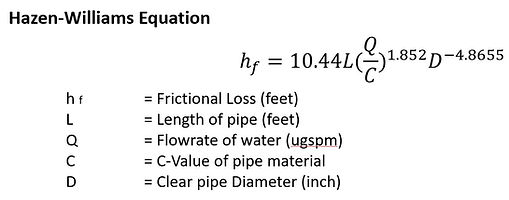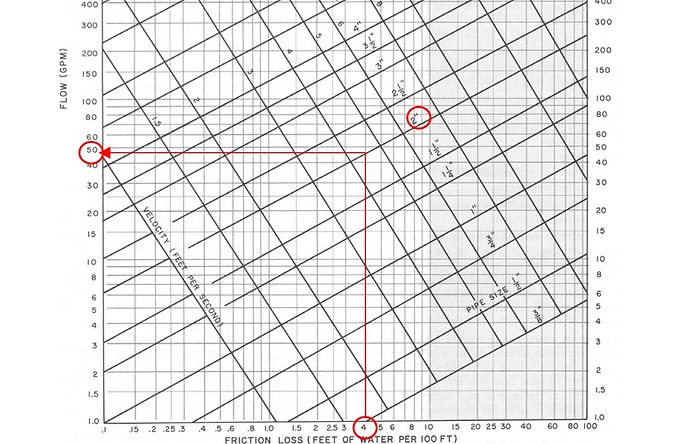top of pagePipe Size Calculator

One of the most renowned methods of pipe sizing is using the Hazen-Williams equation, especially when the fluid involved is water.The equation estimates the frictional loss (feet) for a specific pipe with Length, L (feet), Diameter, D (inch), Flowrate, Q (usgpm) and C-value: Hazen-Williams coefficient, C.

The C-value of different materials of pipes can be referenced from various sources, which are summarized below. The higher the C-value, the “smoother” the flow in the pipe.

We can use the Hazen-Williams equation to estimate pipe sizes, D, required for a given pipe C-value, C, flowrate, Q, and the allowable frictional loss over a fixed 100 feet length of pipe. This "ft/100ft" maximum allowable friction loss is commonly set in the range within 4 to 10ft/100ft. 4ft/100ft means that 4 feet of loss is the maximum that can be accepted in our design per 100 feet run of pipe. This value is then referred in a pipe size chart as shown below. This chart is derived from the Equation for a specific C-value, in this case, for the material of Schedule 40 Pipe, for closed piping system.For example, a 2” Schedule 40 pipe can cater up to 50 usgpm of flow in closed piping system without exceeding 4 feet of frictional loss for every 100 feet run of the pipe.

In order to derive the minimum clear pipe diameter required based on a given maximum allowable loss instead, the equation needs to be modified by making D the subject.The calculator below adapts the formula for a chosen pipe material with its corresponding C-value. You can also download the Excel file with more functions/change of setting in the calculation.

Pipe Size Calculator (Excel)bottom of page